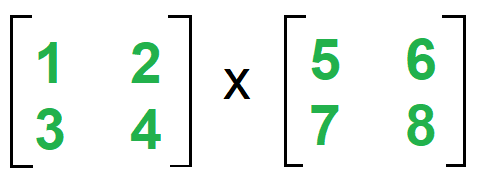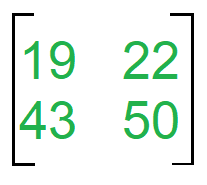Open in App
Not now

# Matrix Multiplication With 1 MapReduce Step

• Difficulty Level : Medium
• Last Updated : 11 Jan, 2023

MapReduce is a technique in which a huge program is subdivided into small tasks and run parallelly to make computation faster, save time, and mostly used in distributed systems. It has 2 important parts:

• Mapper: It takes raw data input and organizes into key, value pairs. For example, In a dictionary, you search for the word “Data” and its associated meaning is “facts and statistics collected together for reference or analysis”. Here the Key is Data and the Value associated with is facts and statistics collected together for reference or analysis.
• Reducer: It is responsible for processing data in parallel and produce final output.

Let us consider the matrix multiplication example to visualize MapReduce. Consider the following matrix:2×2 matrices A and B

Here matrix A is a 2×2 matrix which means the number of rows(i)=2 and the number of columns(j)=2. Matrix B is also a 2×2 matrix where number of rows(j)=2 and number of columns(k)=2. Each cell of the matrix is labelled as Aij and Bij. Ex. element 3 in matrix A is called A21 i.e. 2nd-row 1st column. Now One step matrix multiplication has 1 mapper and 1 reducer. The Formula is:

Mapper for Matrix A (k, v)=((i, k), (A, j, Aij)) for all k
Mapper for Matrix B (k, v)=((i, k), (B, j, Bjk)) for all i

Therefore computing the mapper for Matrix A:

```# k, i, j computes the number of times it occurs.
# Here all are 2, therefore when k=1, i can have
# 2 values 1 & 2, each case can have 2 further
# values of j=1 and j=2. Substituting all values
# in formula

k=1  i=1  j=1   ((1, 1), (A, 1, 1))
j=2   ((1, 1), (A, 2, 2))
i=2  j=1   ((2, 1), (A, 1, 3))
j=2   ((2, 1), (A, 2, 4))

k=2  i=1  j=1   ((1, 2), (A, 1, 1))
j=2   ((1, 2), (A, 2, 2))
i=2  j=1   ((2, 2), (A, 1, 3))
j=2   ((2, 2), (A, 2, 4)) ```

Computing the mapper for Matrix B

```i=1  j=1  k=1   ((1, 1), (B, 1, 5))
k=2   ((1, 2), (B, 1, 6))
j=2  k=1   ((1, 1), (B, 2, 7))
k=2   ((1, 2), (B, 2, 8))

i=2  j=1  k=1   ((2, 1), (B, 1, 5))
k=2   ((2, 2), (B, 1, 6))
j=2  k=1   ((2, 1), (B, 2, 7))
k=2   ((2, 2), (B, 2, 8)) ```

The formula for Reducer is:

Reducer(k, v)=(i, k)=>Make sorted Alist and Blist
(i, k) => Summation (Aij * Bjk)) for j
Output =>((i, k), sum)

Therefore computing the reducer:

```# We can observe from Mapper computation
# that 4 pairs are common (1, 1), (1, 2),
# (2, 1) and (2, 2)
# Make a list separate for Matrix A &
# B with adjoining values taken from
# Mapper step above:

(1, 1) =>Alist ={(A, 1, 1), (A, 2, 2)}
Blist ={(B, 1, 5), (B, 2, 7)}
Now Aij x Bjk: [(1*5) + (2*7)] =19 -------(i)

(1, 2) =>Alist ={(A, 1, 1), (A, 2, 2)}
Blist ={(B, 1, 6), (B, 2, 8)}
Now Aij x Bjk: [(1*6) + (2*8)] =22 -------(ii)

(2, 1) =>Alist ={(A, 1, 3), (A, 2, 4)}
Blist ={(B, 1, 5), (B, 2, 7)}
Now Aij x Bjk: [(3*5) + (4*7)] =43 -------(iii)

(2, 2) =>Alist ={(A, 1, 3), (A, 2, 4)}
Blist ={(B, 1, 6), (B, 2, 8)}
Now Aij x Bjk: [(3*6) + (4*8)] =50 -------(iv)

From (i), (ii), (iii) and (iv) we conclude that
((1, 1), 19)
((1, 2), 22)
((2, 1), 43)
((2, 2), 50)```

Therefore the Final Matrix is:Final output of Matrix multiplication.

My Personal Notes arrow_drop_up
Related Articles